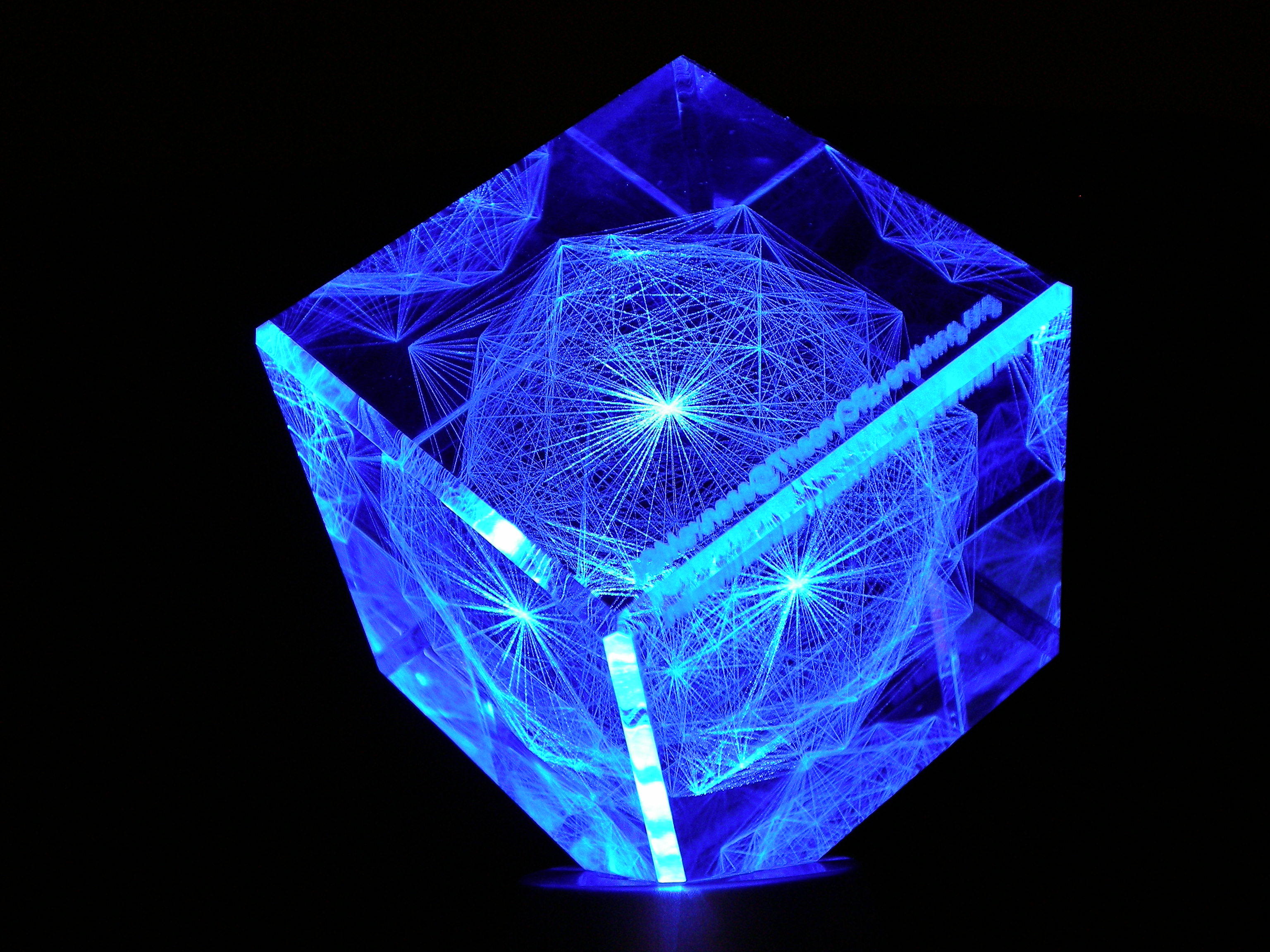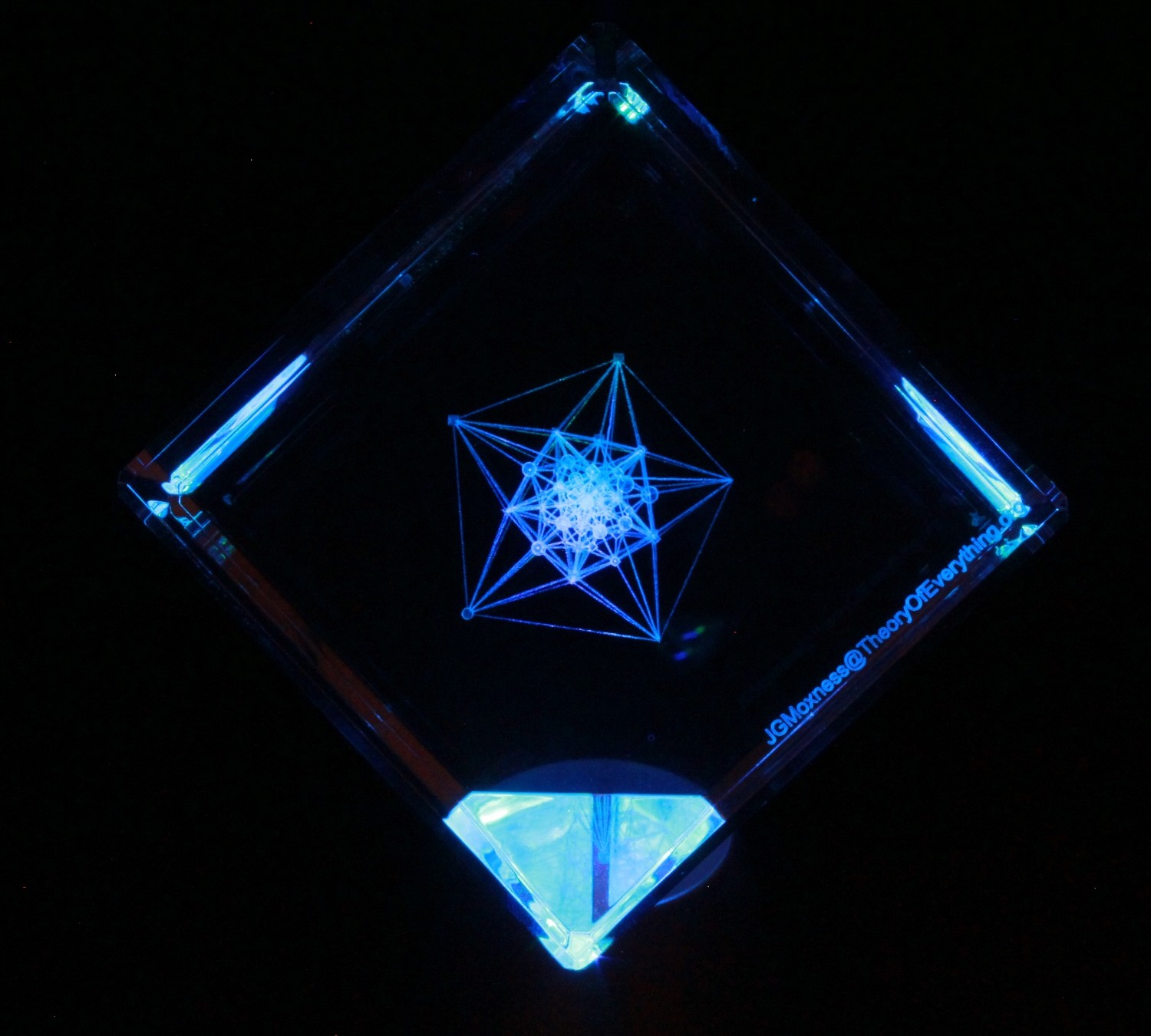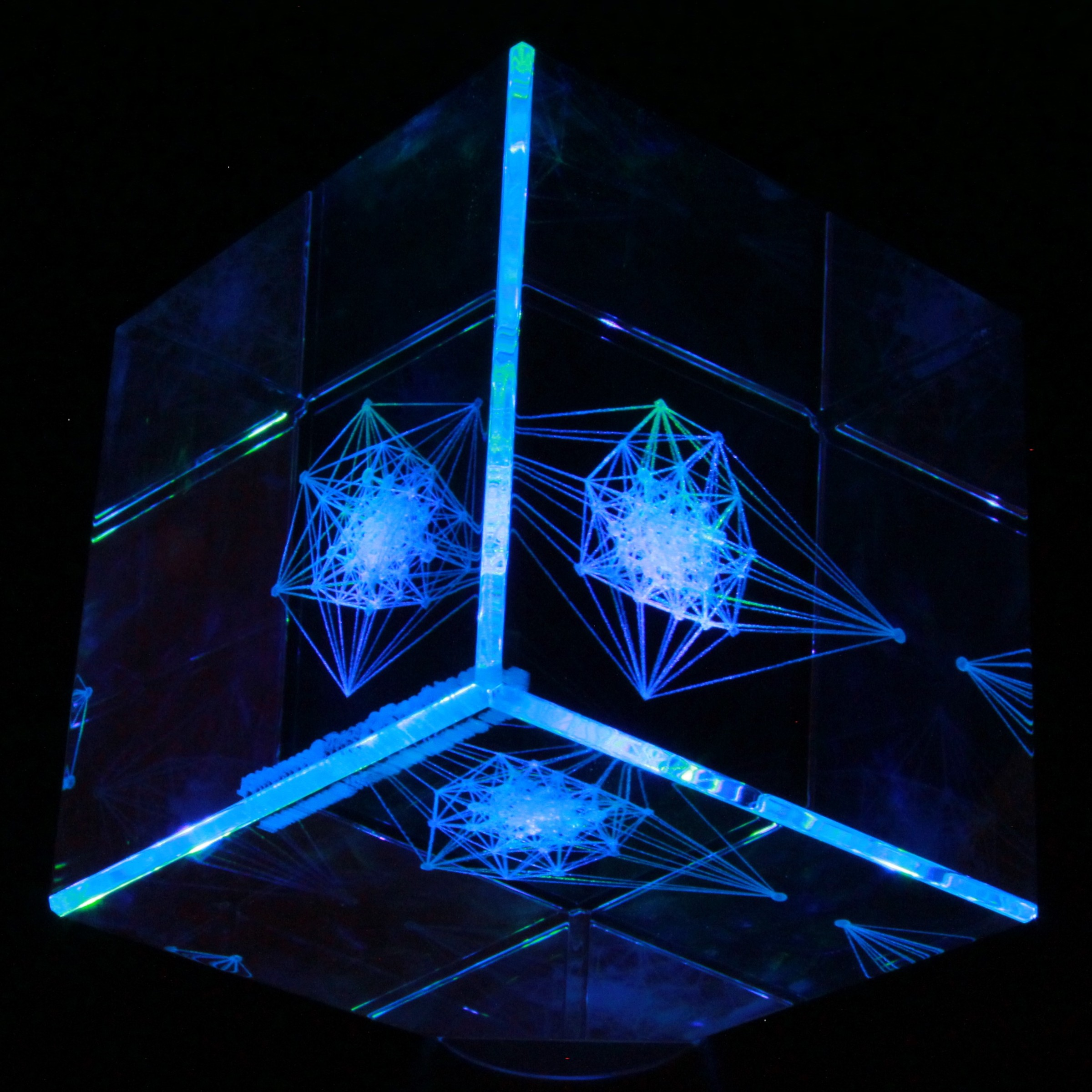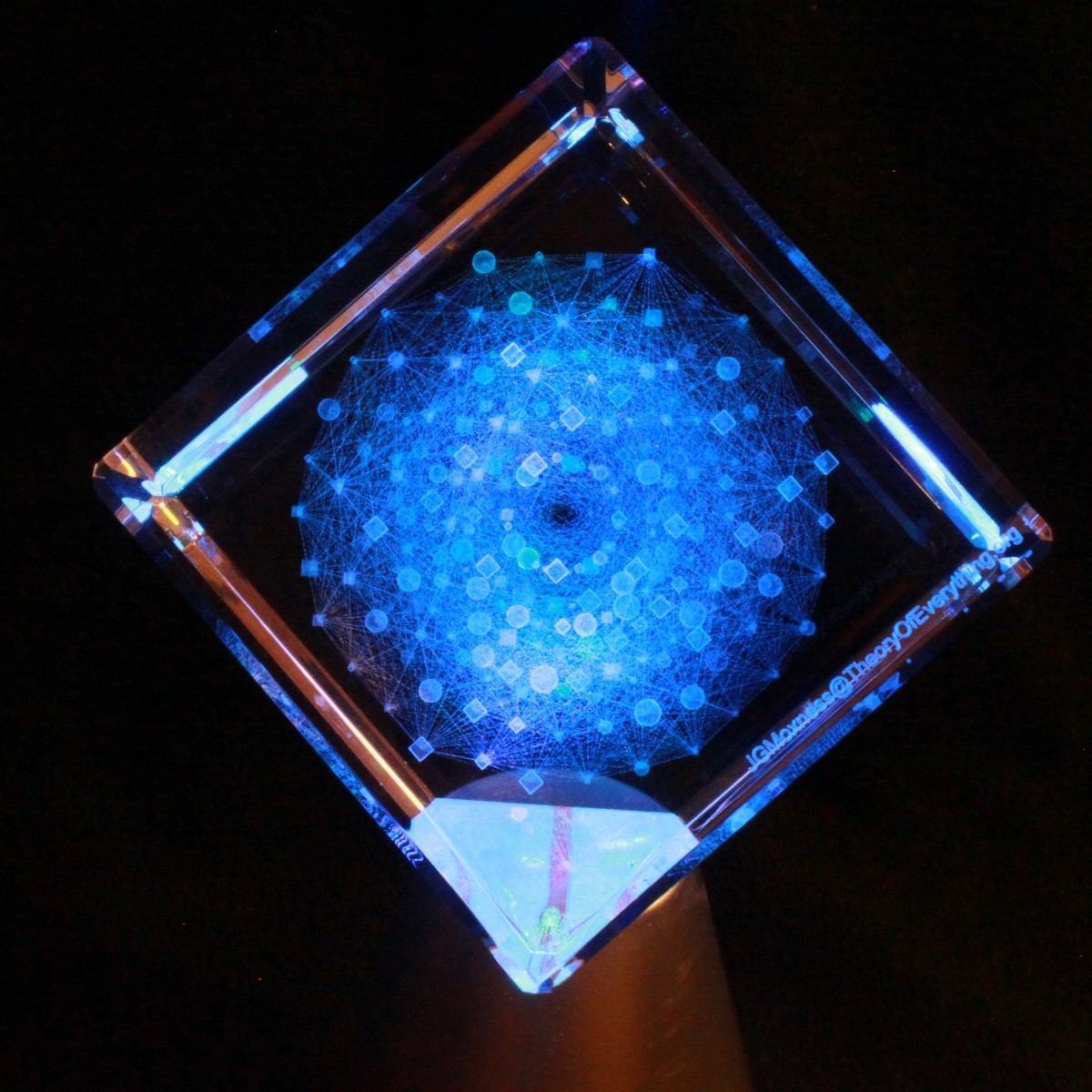# Avengers Tesseract Cosmic Cube

I finally got around to watching the Avengers movie and noticed that the Tesseract Cosmic Cube looked much like the hyper-dimensional projections that I make with laser etched optical crystal.

The blue light is projected from a multi-colored LED base.

While I do have tesseract projections, the E8 projection on my home page seems most similar. Here are a few blue crystal photos I’ve taken that are also similar.# E8 to 4D (3D+T)

E8 8D to 4D (3D+T) Animation

Shown in this animation are the 240 vertices of E8 with shape, size, and color assigned based on theoretical physics of an extended Standard Model (eSM). It is made up of three sets of 120 frames, each with a different algorithm for calculating perspective and orthogonal, rotational and translational 8D flight paths. It is interesting to note that it is the 8D camera that is moving through 8D space and the vertices remain in their same 8D position.

The 30 blue triangles represent E8 triality relationships using an 8D rotation matrix based on 2Pi/3 (or 120 degrees). Each vertex in a blue triangle is transformed into an adjacent one by the dot product with the matrix. A second transformation transforms it to the next, while the third recovers the original vertex.

The 28 red and green triangles are created from a subset of the 6720 (shortest) edges of 8D norm’d length Sqrt(2). These are filtered to represent the particle sums (linked by a red line) for a common (clicked) vertex (linked by 2 green lines). It is interesting to note that all sums for a given vertex are only found in adjacent vertices.

Higher definition (2 sets 60 frames each):